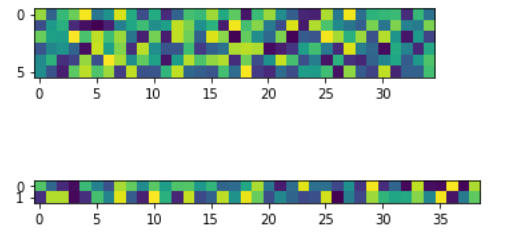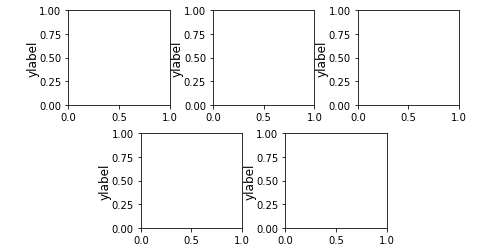# Matplotlib.gridspec.GridSpec Class in Python

• Last Updated : 07 Oct, 2021

Matplotlib is an amazing visualization library in Python for 2D plots of arrays. Matplotlib is a multi-platform data visualization library built on NumPy arrays and designed to work with the broader SciPy stack.

## matplotlib.gridspec.GridSpec

The matplotlib.gridspec.GridSpec class is used to specify the geometry of the grid to place a subplot. For this, to work the number of rows and columns must be set. Optionally, tuning of subplot layout parameters can be also done.

Attention geek! Strengthen your foundations with the Python Programming Foundation Course and learn the basics.

To begin with, your interview preparations Enhance your Data Structures concepts with the Python DS Course. And to begin with your Machine Learning Journey, join the Machine Learning - Basic Level Course

Syntax: class matplotlib.gridspec.GridSpec(nrows, ncols, figure=None, left=None, bottom=None, right=None, top=None, wspace=None, hspace=None, width_ratios=None, height_ratios=None)
Parameters:

• nrows: It is an integer representing the number of rows in the grid.

• ncols: It is an integer representing the number of columns in the grid.

• figure: It is an optional parameter used to draw figures.

• left, right, top, bottom: These are optional parameters used to define the extent of the subplots as fraction of figure width or height.

• wspase: It is an optional float argument used to reserve the width space between subplots.

• hspace: It is an optional float argument used to reserve the height space between subplots.

• width_ratios: It is an optional parameter that represents the width ratios of the columns.

• height_ratios: It is an optional parameter that represents the width ratios of the rows.

Methods of the class:

• get_subplot_params(self, figure=None): It returns a dictionary of subplot layout parameters. unless a figure attribute is set, the default parameter is from rcParams.

Example 1:

## Python3

 `import` `numpy as np``import` `matplotlib.pyplot as plt``from` `matplotlib.gridspec ``import` `GridSpec`  `gs ``=` `GridSpec(``8``, ``39``)``ax1 ``=` `plt.subplot(gs[:``6``, :``35``])``ax2 ``=` `plt.subplot(gs[``6``:, :])` `data1 ``=` `np.random.rand(``6``, ``35``)``data2 ``=` `np.random.rand(``2``, ``39``)` `ax1.imshow(data1)``ax2.imshow(data2)` `plt.show()`

Output:Example 2:

## Python3

 `import` `matplotlib.pyplot as plt``import` `matplotlib.gridspec as gridspec`  `fig ``=` `plt.figure(figsize ``=``([``7``, ``4``]))` `gs ``=` `gridspec.GridSpec(``2``, ``6``)``gs.update(wspace ``=` `1.5``, hspace ``=` `0.3``)` `ax1 ``=` `plt.subplot(gs[``0``, :``2``])``ax1.set_ylabel(``'ylabel'``, labelpad ``=` `0``, fontsize ``=` `12``)` `ax2 ``=` `plt.subplot(gs[``0``, ``2``:``4``])``ax2.set_ylabel(``'ylabel'``, labelpad ``=` `0``, fontsize ``=` `12``)` `ax3 ``=` `plt.subplot(gs[``0``, ``4``:``6``])``ax3.set_ylabel(``'ylabel'``, labelpad ``=` `0``, fontsize ``=` `12``)` `ax4 ``=` `plt.subplot(gs[``1``, ``1``:``3``])``ax4.set_ylabel(``'ylabel'``, labelpad ``=` `0``, fontsize ``=` `12``)` `ax5 ``=` `plt.subplot(gs[``1``, ``3``:``5``])``ax5.set_ylabel(``'ylabel'``, labelpad ``=` `0``, fontsize ``=` `12``)` `plt.show()`

Output:My Personal Notes arrow_drop_up